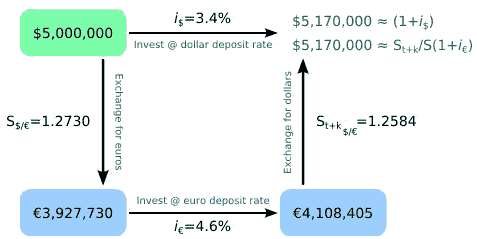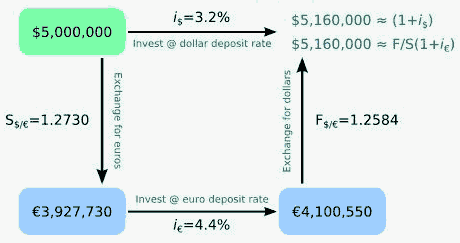# Interest Rate Parity Model

The Model of Interest Rate Parity states that in the event that two different currencies have different interest rates, then that difference will be reflected in the premium or discount to the price in the future, in order to avoid arbitration without risks.

For example, if interest rates in the U.S. are 3% and the interest rates of Japan are 1%, then the U.S. dollar (USD) should depreciate against the Japanese yen (JPY) by 2% to avoid what is known as risk arbitrage. This price or future exchange rate is expressed in the price at future date (forward) indicated on the current date.

In this example, it is said that the price or forward exchange rate for the U.S. dollar is at a discount because this currency buys fewer yens in the forward price that in the spot price. Therefore, it is said that the Japanese yen is at a premium.

## Description

The Interest rate parity is a theory that describes the relationship between exchange rates between currencies and interest rates.

The interest rate parity represents an equilibrium state in which the expected benefit, expressed in national currency, is the same for assets denominated in national currency and assets denominated in foreign currency of similar risk and term, provided that arbitration is not made. This is because the exchange rate in the currency market between both currencies balances the return of both investments. According to the interest rate parity theory, several situations can be found that we will see below: uncovered interest rate parity and covered interest rate parity.

### Assumptions

The interest rate parity theory starts from several assumptions as a basis:

• Mobility of capital: the investor’s capital can be easily exchanged between domestic and foreign assets.
• Perfect replacement: national and foreign assets with similar risk and term are totally substitutable.

Based on these assumptions, an investor will choose the investment in assets with the highest expected profit regardless of whether he is a national or a foreigner investor. This does not mean that a foreign investor and a national investor obtain an equivalent benefit, but that each investor will obtain an equivalent benefit regardless of their decision to invest in a national or foreign asset.

### Uncovered interest rate parity

When the condition of non-arbitration is fulfilled without the use of a forward (term contract) to protect against exposure to foreign exchange risk, it is said that the interest rate parity is uncovered. The exchange rate between the national currency and the foreign currency will adjust the benefit so that the profitability in the national currency of deposits in national currency will be equal to the profitability in the national currency of foreign deposits. The uncovered interest rate parity helps explain the relationship with the spot exchange rate through the following equation:

(1 + in) = ((E(St+k)) / St)(1 + ie)

where:

E (St + k) is the expected future spot exchange rate at time t + k

k is the number of periods in the future since time t

St is the exchange rate in effect at time t

in is the national interest rate

ie is the interest rate abroad

The first part of the equation, 1 + in, is the profitability in the national currency of deposits in national currency. The second part of the equation, ((E (St + k))/St) (1 + ie), is the profitability in the national currency of foreign currency deposits. Thus, the exchange rate is related to the interest rate in both countries.

Diagram of representation of uncovered interest rate parity (image by John Shandy):### Covered interest rate parity

Unlike in the previous case, when the condition of non-arbitration is met together with the use of a forward to hedge against the exposure to foreign exchange risk, it is said that the interest rate parity is covered. The expected benefit will remain the same, for risky assets and similar terms, regardless of whether they are denominated in national currency or in foreign currency. The covered interest rate parity relates the future exchange rate (forward) and interest rates, unlike the uncovered interest rate parity that relates the spot exchange rate and the interest rates.

The following equation represents the covered interest rate parity:

(1 + in) = (Ft/St)(1 + ie)

where:

Ft is the term exchange rate at time t

St is the spot exchange rate in effect at time t

The profitability of deposits denominated in national currency, 1 + in, is equal to the profitability of deposits denominated in foreign currency, (Ft/St) (1 + ie).

Example diagram of covered interest rate parity (image by John Shandy):## Conclusion

In recent years, the parity of interest rates has not produced the best results. This is because usually, the currencies with higher interest rates increase as a result of the decisions of central banks, which try to reduce the rate of an economy that is excessively revolutionized by the rise in interest rates. This type of action taken by central banks has no relation with risk arbitrage.